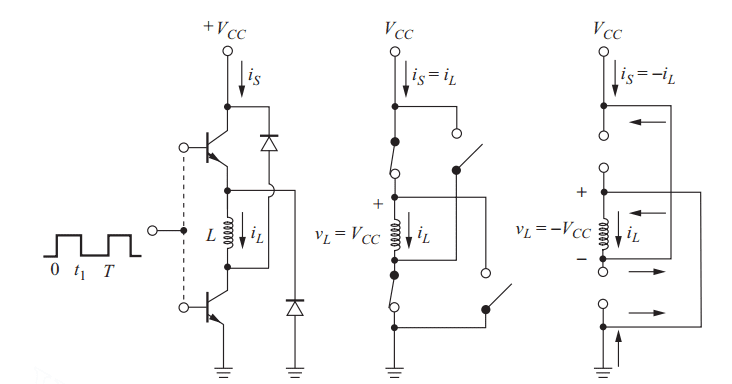# Inductor current calculation

Gold Member
Homework Statement:
An inductor is energized as in the circuit of Fig. The circuit has L = 50 mH, VCC= 90 V, t1= 4 ms, and T=50 ms. (a) Determine the peak energy stored in the inductor. (b) Graph the inductor current, source current, inductor instantaneous power, and source instantaneous power versus time. Assume the transistors are ideal.
Relevant Equations:
P=V*I Watts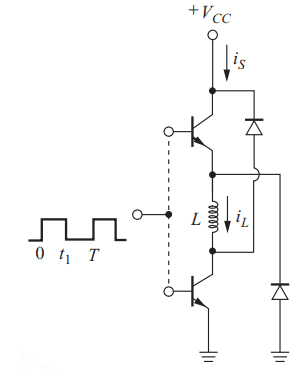a. When the transistors are ON.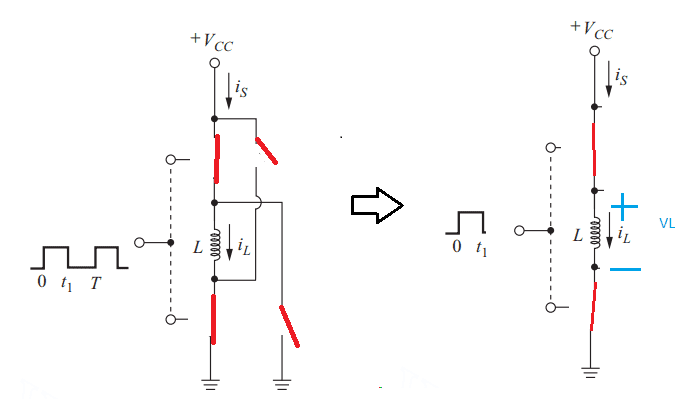##V_{CC} = V_L##
##V_{CC} = L \frac{di_L} {dt} ##
##i_L = \frac{V_{CC}t} L ##

b. I am bit confused when the transistor is "OFF"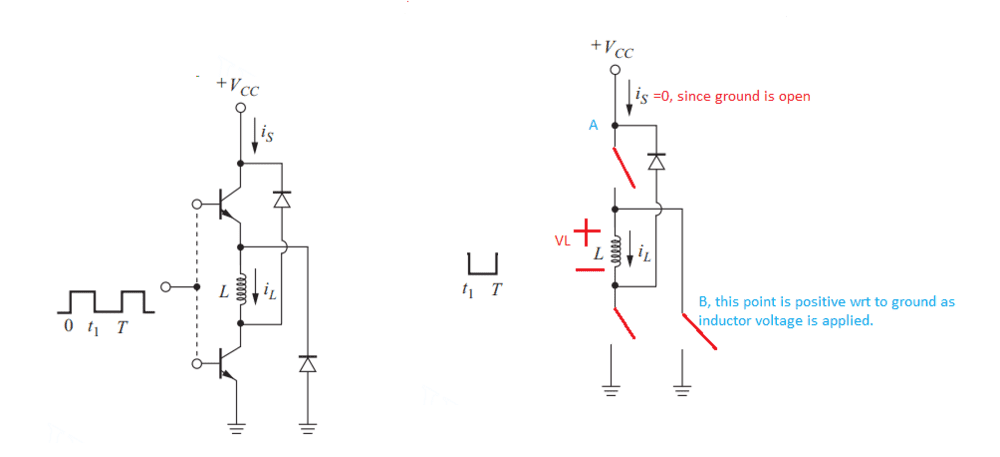The point "A" is floating? The transistors and the diodes all the elements seem to be open is it correct? I am confused here.

•Delta2

DaveE
Gold Member
Yes, you are a bit confused. If you include the power source, the inductor current must flow in a loop at all times (actually true for all currents, since we don't make electrons from nothing). You can't disconnect the inductor from some path for the current flow unless the inductor current is exactly zero. This is because the inductor will generate an induced voltage to maintain the continuity of the current flow. V = L⋅(di/dt), so interrupting the inductor current requires an infinite di/dt, and thus an infinite voltage. Passively switched (commutating) devices are usually controlled by the induced voltage from energy stored in the inductor current.

•PhysicsTest
Gold Member
Thank you for the support,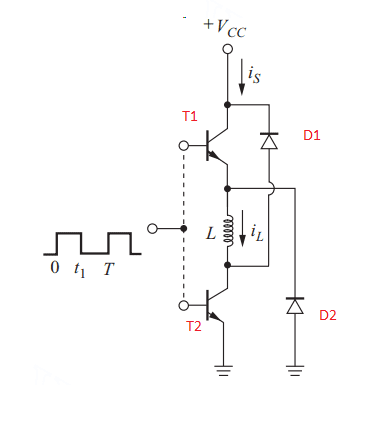Case: 0<t<t1
The applied voltage to the base of the transistor is positive. Hence
T1 = ON,
T2 = ON,
D1, Cathode=##V_{CC}## Volts is applied, Anode, Negative of inductor voltage is applied, hence it is reverse biased and open.
D2, Cathode is positive of inductor voltage and Anode it is grounded, hence it is reverse biased.

The inductor is charged to the voltage say ##V_L ##

Case: t1<t<T
The applied voltage to the base of the transistor is negative. Hence
T1 = OFF,
T2 = OFF,
D1 = OFF, (?)
D2 = ON
The inductor to maintain the continuity of current flow D2 will be ON, the inductor voltage polarity is reversed. One other mistake from my previous post the ##V_{CC}## power source is not completely disconnected as it can be connected through D2 ground. Is the analysis correct? Please help.

DaveE
Gold Member
Thank you for the support,

View attachment 278793
Case: 0<t<t1
The applied voltage to the base of the transistor is positive. Hence
T1 = ON,
T2 = ON,
D1, Cathode=##V_{CC}## Volts is applied, Anode, Negative of inductor voltage is applied, hence it is reverse biased and open.
D2, Cathode is positive of inductor voltage and Anode it is grounded, hence it is reverse biased.

The inductor is charged to the voltage say ##V_L ##

Case: t1<t<T
The applied voltage to the base of the transistor is negative. Hence
T1 = OFF,
T2 = OFF,
D1 = OFF, (?)
D2 = ON
The inductor to maintain the continuity of current flow D2 will be ON, the inductor voltage polarity is reversed. One other mistake from my previous post the ##V_{CC}## power source is not completely disconnected as it can be connected through D2 ground. Is the analysis correct? Please help.
No, case 2 isn't right. If both D1 and T2 are off how will the current flow out of the inductor? Where will it go?

Perhaps when the transistors are off, the inductor has a emf big enough to create a closed loop the other way, upwards. This can happen if ##emf > Vcc##
Homework Statement:: An inductor is energized as in the circuit of Fig. The circuit has L = 50 mH, VCC= 90 V, t1= 4 ms, and T=50 ms. (a) Determine the peak energy stored in the inductor. (b) Graph the inductor current, source current, inductor instantaneous power, and source instantaneous power versus time. Assume the transistors are ideal.
Relevant Equations:: P=V*I Watts

View attachment 278761
a. When the transistors are ON.

View attachment 278762
##V_{CC} = V_L##
##V_{CC} = L \frac{di_L} {dt} ##
##i_L = \frac{V_{CC}t} L ##

b. I am bit confused when the transistor is "OFF"

View attachment 278763
The point "A" is floating? The transistors and the diodes all the elements seem to be open is it correct? I am confused here.

Gold Member
Still trying to figure out the solution, i refreshed the basics of the inductor,
Case1: The Peak current is
##i_L = \frac{90*4} {50} A = 7.2A ##
The voltage across the inductor is constant equal to ##V_{CC} = 90V## during the "ON" time.

In case 2 for the transistor OFF, the inductor will try to maintain the current ##i_L## and in the same direction the circuit will be like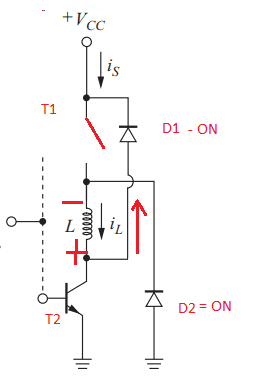I still don't understand why the transistor T2 is "ON"? And also will ##V_{CC}## will be part of the circuit in this case? The circuit is very confusing.

Case 2 looks good, edit: I think I can see now the high potential below ##L## means it's possible for a closed loop: L->T2->D2->L.

Still trying to figure out the solution, i refreshed the basics of the inductor,
Case1: The Peak current is
##i_L = \frac{90*4} {50} A = 7.2A ##
The voltage across the inductor is constant equal to ##V_{CC} = 90V## during the "ON" time.

In case 2 for the transistor OFF, the inductor will try to maintain the current ##i_L## and in the same direction the circuit will be like
View attachment 278869
I still don't understand why the transistor T2 is "ON"? And also will ##V_{CC}## will be part of the circuit in this case? The circuit is very confusing.

Last edited:
Gold Member
Case 2 looks good, edit: I think I can see now the high potential below ##L## means it's possible for a closed loop: L->T2->D2->L.
So you mean T2 will be ON but the input base voltage to the transistor is low it means the transistor T2 should be cutoff? What am i missing?

DaveE
Gold Member
The base drive to the two transistors is simplified to the point that you can only conclude their intent that those switches are on or off according to the waveform sketch. That part isn't a realistic circuit, hence the dashed lines. Just assume that T1 and T2 are on or off at the same time according to the drive waveform shown.

DaveE
Gold Member
I think you're having a hard time understanding that the inductor can generate and induced voltage across itself in order to maintain constant current flow. Remember v = L(di/dt). The only way to get large di/dt in an inductor is to have a large voltage applied to it.

•PhysicsTest
Gold Member
One final question is i don't see a resistor in the circuit for the inductor to discharge. I assume that a resistor is mandatory to discharge?

DaveE
•